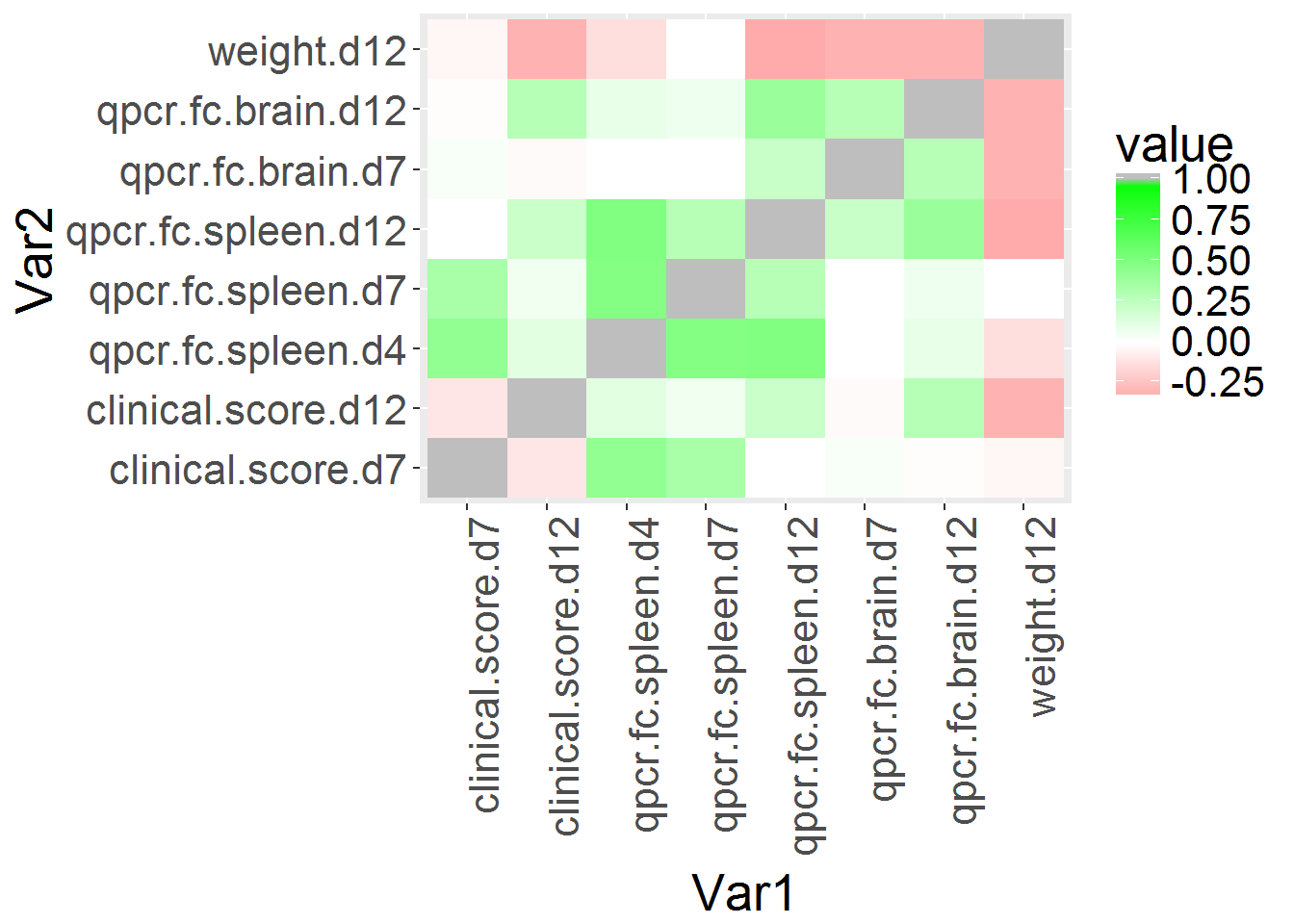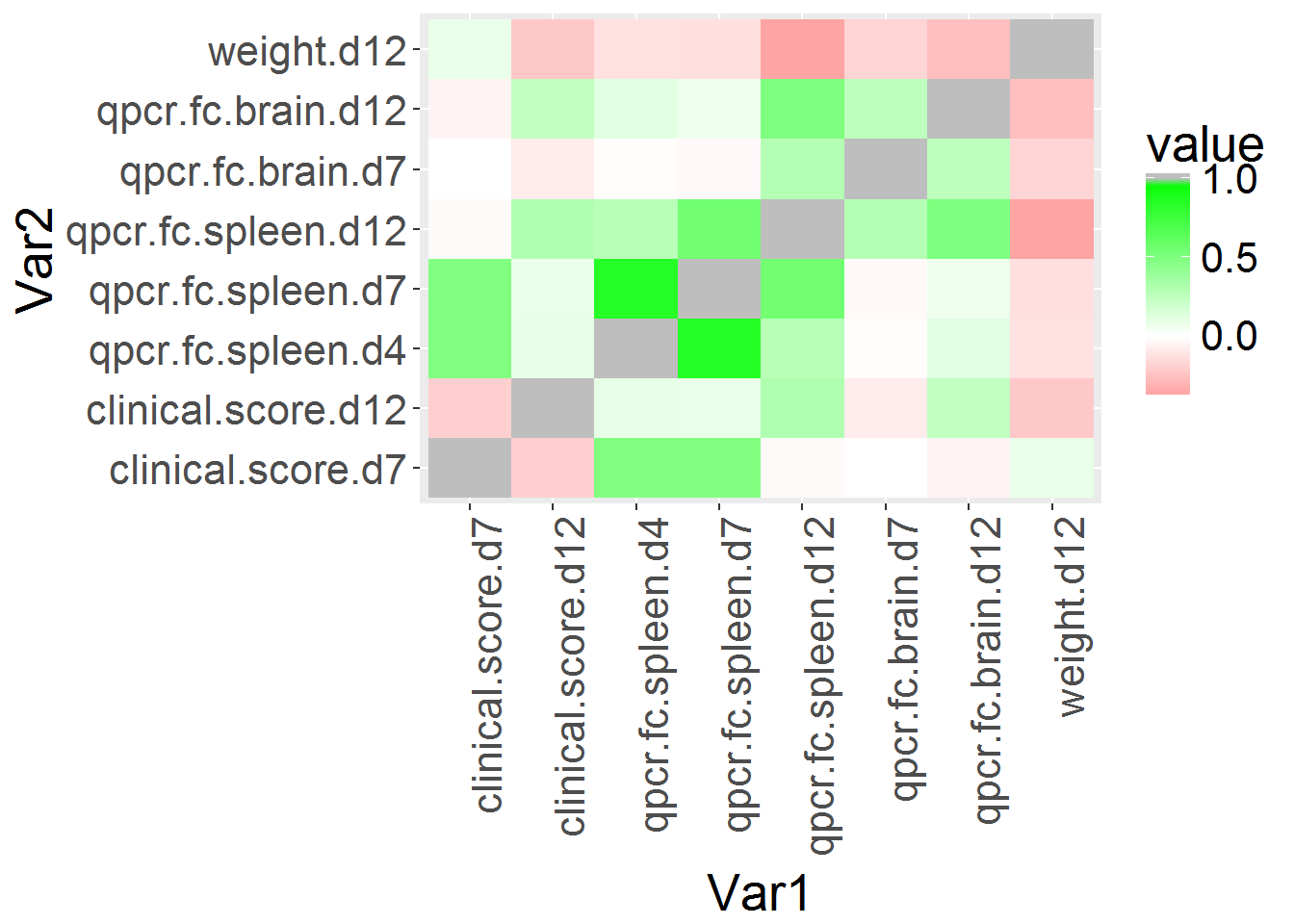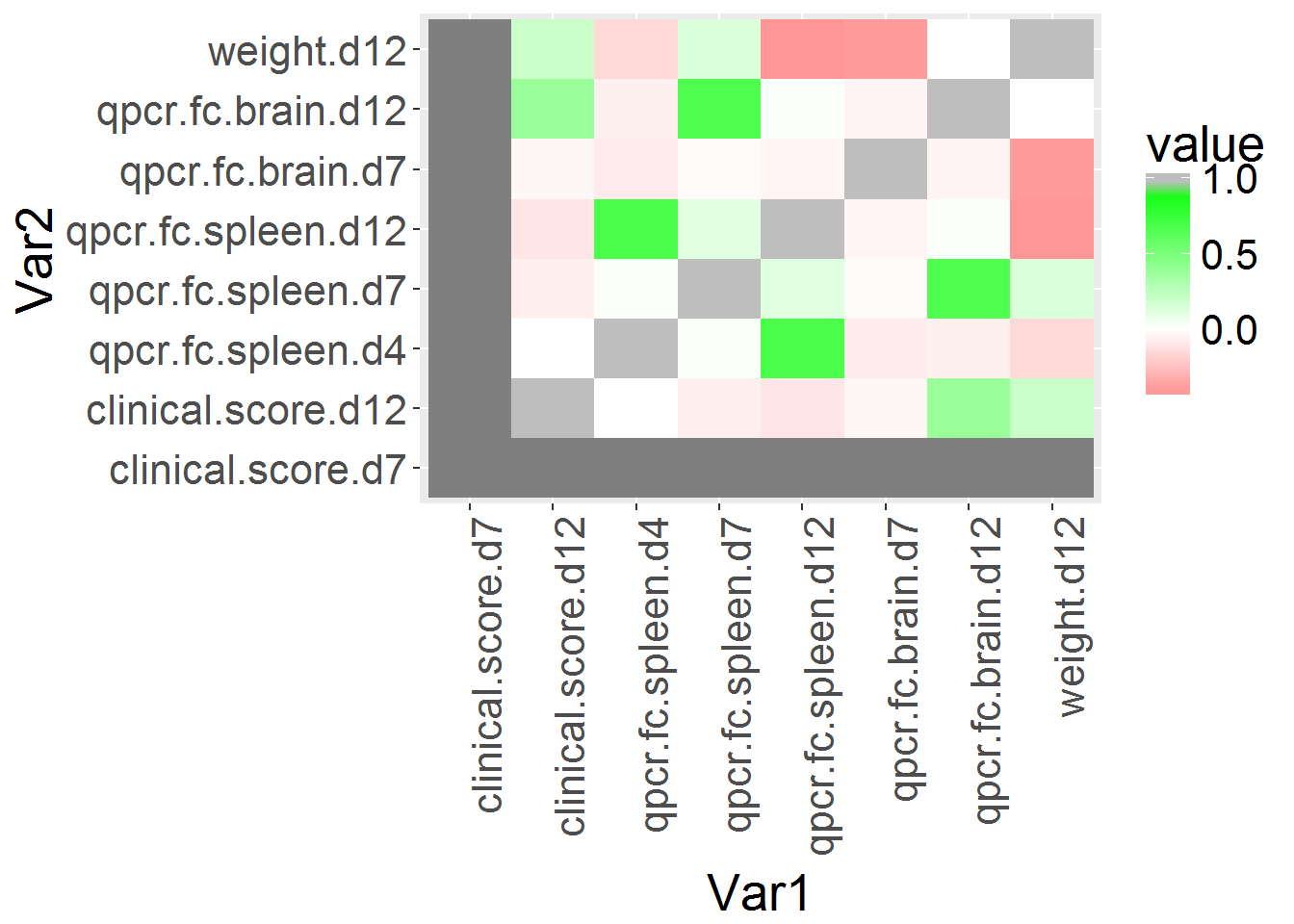This is a data report outlining the a pearson correlation analysis of viral qpcr and clinical score data in the CC lines.

This analysis is based off code documented here:

https://www.r-bloggers.com/r-defining-your-own-color-schemes-for-heatmaps/

library(gplots)
## Warning: package 'gplots' was built under R version 3.2.5
##
## Attaching package: 'gplots'
## The following object is masked from 'package:stats':
##
##     lowess
library(ggplot2)
library(reshape2)
## Warning: package 'reshape2' was built under R version 3.2.5
sessionInfo()
## R version 3.2.3 (2015-12-10)
## Platform: x86_64-w64-mingw32/x64 (64-bit)
## Running under: Windows 10 x64 (build 14393)
##
## locale:
##  LC_COLLATE=English_United States.1252
##  LC_CTYPE=English_United States.1252
##  LC_MONETARY=English_United States.1252
##  LC_NUMERIC=C
##  LC_TIME=English_United States.1252
##
## attached base packages:
##  stats     graphics  grDevices utils     datasets  methods   base
##
## other attached packages:
##  reshape2_1.4.2 ggplot2_2.2.1  gplots_3.0.1
##
## loaded via a namespace (and not attached):
##   Rcpp_0.12.8        knitr_1.15.1       magrittr_1.5
##   munsell_0.4.3      colorspace_1.3-2   stringr_1.1.0
##   plyr_1.8.4         caTools_1.17.1     tools_3.2.3
##  grid_3.2.3         gtable_0.2.0       KernSmooth_2.23-15
##  htmltools_0.3.5    gtools_3.5.0       assertthat_0.1
##  lazyeval_0.2.0     yaml_2.1.14        rprojroot_1.1
##  digest_0.6.11      tibble_1.2         bitops_1.0-6
##  evaluate_0.10      rmarkdown_1.3      gdata_2.17.0
##  stringi_1.1.2      scales_0.4.1       backports_1.0.4
oas1b_corr_plot <- read.csv(file="C:\\gale_lab\\oas1b_manuscript\\revisions\\qPCR_and_outcomes.csv", header=T)

#transponse values
m <- melt(cor(oas1b_corr_plot[,c(5:12)], use="pairwise.complete.obs"))

# create a correlation plot image using ggplot2
p <- ggplot(data=m, aes(x=Var1, y=Var2, fill=value)) + geom_tile() + theme(text = element_text(size=20),
axis.text.x = element_text(angle=90, hjust=1))

We update the color panel to show those conditions with the highest correlation.

#set up a coloring scheme using colorRampPalette
red=rgb(1,0,0); green=rgb(0,1,0); blue=rgb(0,0,1); white=rgb(1,1,1)
RtoWrange<-colorRampPalette(c(red, white ) )
WtoGrange<-colorRampPalette(c(white, green) )

p <- p + scale_fill_gradient2(low=RtoWrange(100), mid=WtoGrange(100), high="gray")

p + theme(text = element_text(size=20),
axis.text.x = element_text(angle=90, hjust=1))In the correlation heatmap above, there is a relationship between viral load and clinical score at days 4 and 7 post infection. There is also a correlation between clinical score and viral load in the brain at day 12 post infection.

But this is against all Oas1b haplotypes, lets see if we observe a correlation in the Null/Null lines (N/N) and Heterozygous lines.

library(dplyr)
## Warning: package 'dplyr' was built under R version 3.2.5
##
## Attaching package: 'dplyr'
## The following objects are masked from 'package:stats':
##
##     filter, lag
## The following objects are masked from 'package:base':
##
##     intersect, setdiff, setequal, union
#filter heterozygous data

oas1b_corr_plot_NF_FN <- filter(oas1b_corr_plot, Oas1b_status %in% c("Null+Functional","Functional+Null"))

m_NF_FN <- melt(cor(oas1b_corr_plot_NF_FN[,c(5:12)],use="pairwise.complete.obs"))
## Warning in cor(oas1b_corr_plot_NF_FN[, c(5:12)], use =
## "pairwise.complete.obs"): the standard deviation is zero
# filter null data

oas1b_corr_plot_NN <- filter(oas1b_corr_plot, Oas1b_status %in% c("Null+Null"))

#plot null null data

m_NN <- melt(cor(oas1b_corr_plot_NN[,c(5:12)],use="pairwise.complete.obs"))

p_NN <- ggplot(data=m_NN, aes(x=Var1, y=Var2, fill=value)) + geom_tile() + theme(text = element_text(size=20),
axis.text.x = element_text(angle=90, hjust=1))

#reset the coloring scheme using colorRampPalette
red=rgb(1,0,0); green=rgb(0,1,0); blue=rgb(0,0,1); white=rgb(1,1,1)
RtoWrange<-colorRampPalette(c(red, white ) )
WtoGrange<-colorRampPalette(c(white, green) )

p_NN <- p_NN + scale_fill_gradient2(low=RtoWrange(100), mid=WtoGrange(100), high="gray")

p_NN + theme(text = element_text(size=20),
axis.text.x = element_text(angle=90, hjust=1))We note a higher correlation with the clinical score and disease phenotypes in N/N RIXs.

Now lets looks at heterzygous RIXs and their relationships to phenotypes

p_NF_FN <- ggplot(data=m_NF_FN, aes(x=Var1, y=Var2, fill=value)) + geom_tile() + theme(text = element_text(size=20),
axis.text.x = element_text(angle=90, hjust=1))

#reset the coloring scheme using colorRampPalette
red=rgb(1,0,0); green=rgb(0,1,0); blue=rgb(0,0,1); white=rgb(1,1,1)
RtoWrange<-colorRampPalette(c(red, white ) )
WtoGrange<-colorRampPalette(c(white, green) )

p_NF_FN <- p_NF_FN + scale_fill_gradient2(low=RtoWrange(100), mid=WtoGrange(100), high="gray")

p_NF_FN + theme(text = element_text(size=20),
axis.text.x = element_text(angle=90, hjust=1))We note a lower correlation (but still observable) with the clinical score and disease phenotypes in F/N and N/F RIXs.